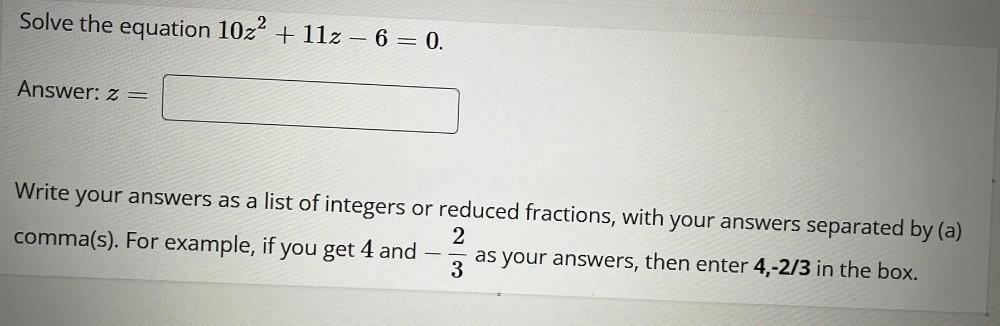Question:

# Solve the equation 10z^2 + 11z – 6 = 0. Write your answers

Last updated: 7/26/2022Solve the equation 10z^2 + 11z – 6 = 0. Write your answers as a list of integers or reduced fractions, with your answers separated by (a) comma(s). For example, if you get 4 and -2/3 as your answers, then enter 4,-2/3 in the box.